|

# 四个关键词参透新中式风格家装秘笈

中国有句老话“三十年河东三十年河西”。在设计师们眼里，时尚就是一股轮回进化的风潮。在中国文化风靡全球的今天，东方美学焕发新的生机。这不，已有粉丝点名让乐活君聊聊“新中式风格的装修”。乐活君不禁感慨：等到风景都看透，还是老祖宗留下的东西最有看头。不信？看看下面的案例再做定夺也不迟啊。

话说，新中式风格不是纯粹的元素堆砌，而是通过对传统文化的认识，将现代元素和传统元素结合在一起，以现代人的审美需求来打造富有传统韵味的事物，让传统艺术的脉络得以传承。 “中式虽好，就是太老”这样的观念套在新中式风格上可就不合适咯。跟着乐活君锻炼一下自己的混搭能力，四个关键词参透新中式风格家装秘笈。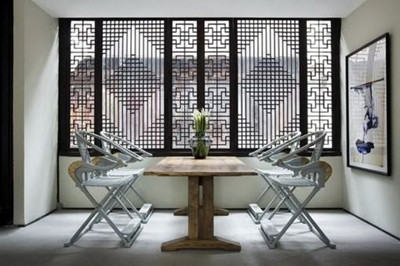关键词一：文化

任何一种风格演绎都有其特定的文化背景作为支撑。新中式是以中国传统古典文化为背景 ，营造富有中国特色的浪漫情调。瓷器、陶艺、中式窗花、字画、布艺以及具有一定含义的中式古典物品等等都体现了浓郁的东方之美，这正是新中式风格与其它风格所不同的地方。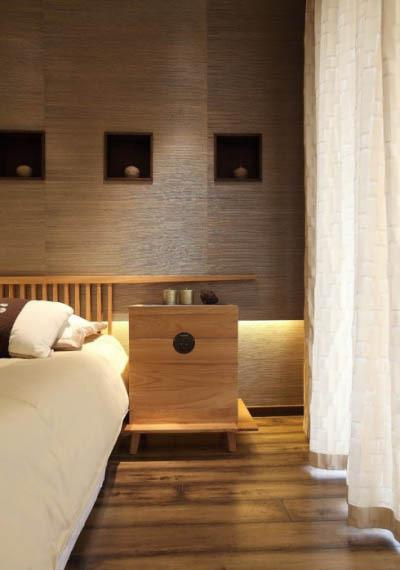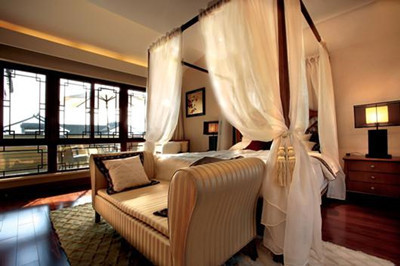新中式风格是中国传统风格文化意义在当前时代背景下的演绎，是对中国当代文化充分理解基础上的当代设计。中国传统的室内设计融合了庄重与优雅双重气质。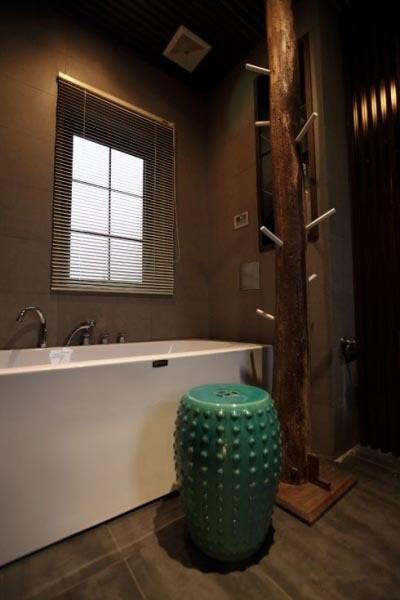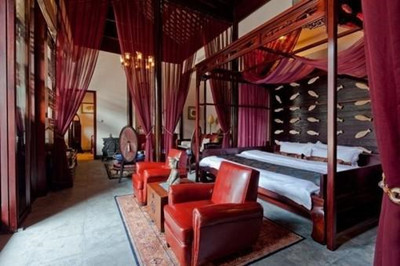现在的中式风格更多地利用了后现代手法，把传统的结构形式通过重新设计组合以另一种民族特色的标志符号出现。例如，厅里摆一套明清式的红木家具，墙上挂一幅中国山水画等，传统的书房里自然少不了书柜、书案以及文房四宝。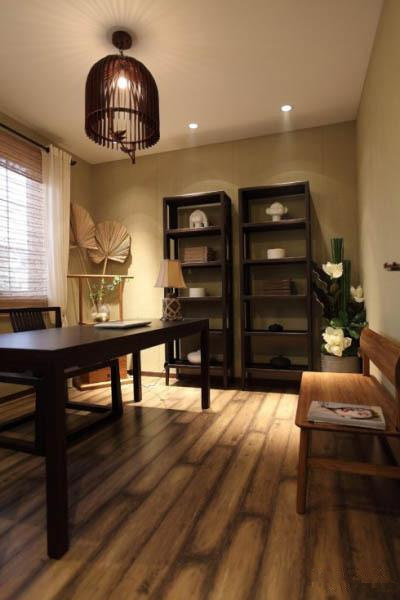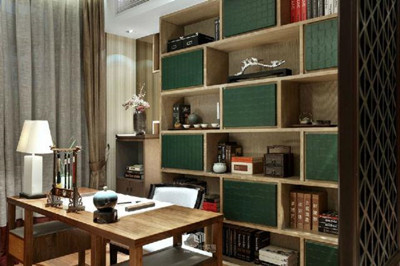中国风的构成主要体现在传统家具（多为明清家具为主）、装饰品及黑、红为主的装饰色彩上。室内多采用对称式的布局方式，格调高雅，造型简朴优美，色彩浓重而成熟。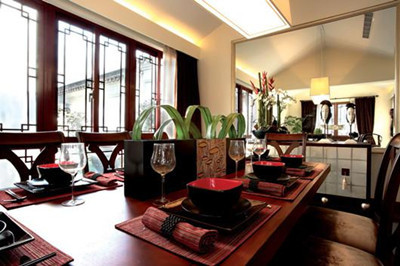关键词二：层次

新中式风格非常讲究空间的层次感与跳跃感。使用中式的屏风、窗棂、中式木门、博古架等进行视觉分割。将单元式住宅就展现出中式家居的层次之美。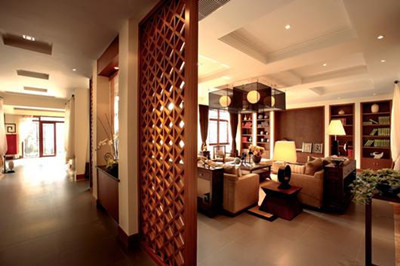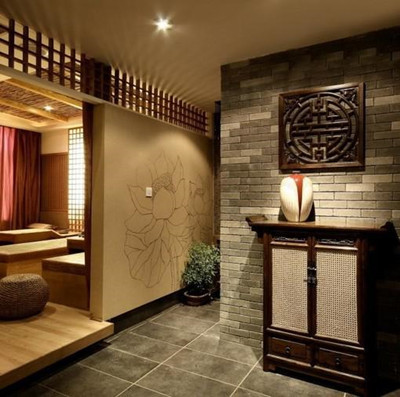中式元素与现代材质的巧妙兼柔，明清家具、窗棂、布艺床品相互辉映，再现了移步变景的精妙小品。配以简约的造型为基础，添加了中式元素，使整体空间更加丰富，大而不空、厚而不重，有格调又不显压抑。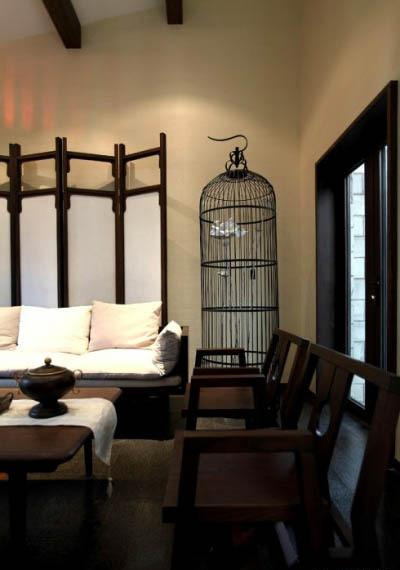继承明清时期家居理念的精华，将其中的经典元素提炼并加以丰富，同时改变原有空间布局中等级、尊卑等封建思想，给传统家居文化注入了新的气息。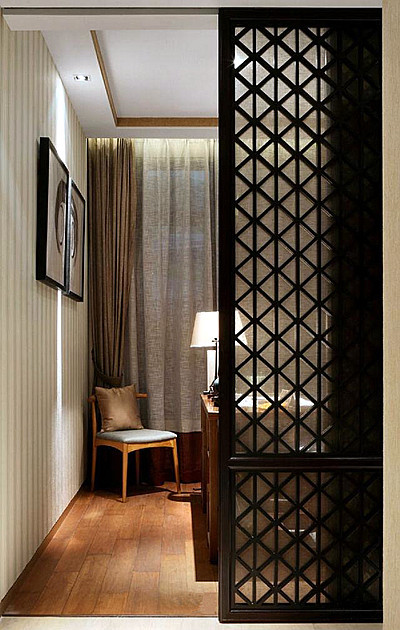关键词三：线条

新中式设计讲究线条简单流畅、融合着精雕细琢的意识。与传统中式家具最大的不同就是，虽有传统元素的神韵，却不是一味照搬。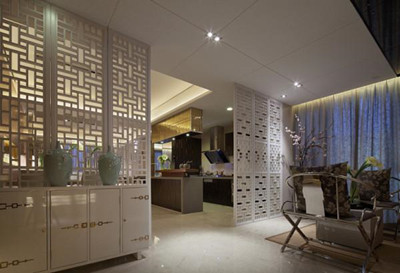传统文化中的象征性元素，如中国结、山水字画、青花瓷、花卉、如意、瑞兽、“回”字纹、波浪形等，常常出现在新中式家具。但是造型更为简洁流畅，雕刻图案将简洁与复杂巧妙地融合，既透露着浓厚的自然气息，又体现出巧夺天工的精细。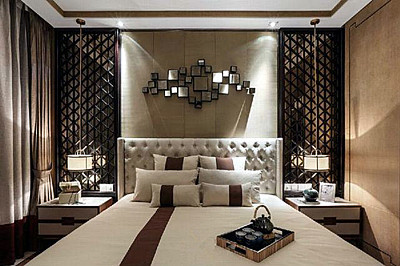空间装饰多采用简洁硬朗的直线条。直线装饰在空间中的使用，不仅反映出现代人追求简单生活的居住要求，更迎合了中式家具追求内敛、质朴的设计风格，使“新中式”更加实用、更富现代感。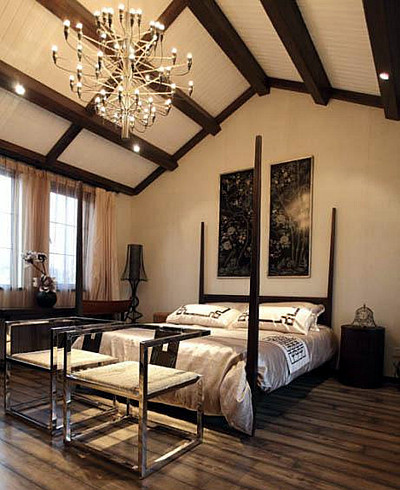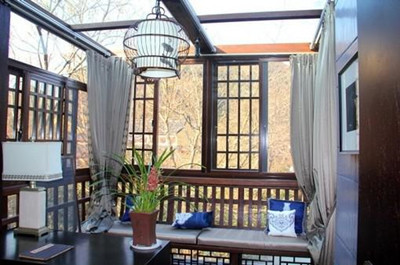关键词四：实用

部分明式坐具的线条过分横平竖直，与人体的腰背部曲线难以贴合。清式坐具背部繁复的雕花，让人感觉很不舒服。但是新中式家具在这方面作出了重大的改革。如沙发扶手、背靠、椅子的靠背、座板等，融入了科学的人体工学设计，具有严谨的结构和线条，沙发坐垫部分的填充物偏软，靠背部的偏硬，加上特制的腰枕，贴合人体曲线，更具人性化设计。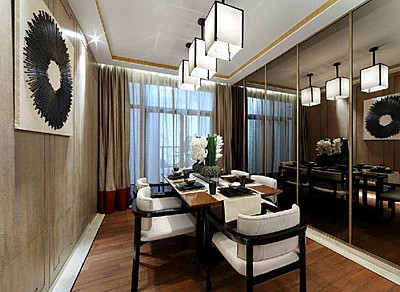另外中式风格的客厅具有内蕴的风格，为了舒服，中式的环境中也常常用到沙发，但颜色仍然体现着中式的古朴，中式风格这种表现使整个空间，传统中透着现代，现代中揉着古典。这样就以一种东方人的“留白”美学观念控制的节奏，显出大家风范。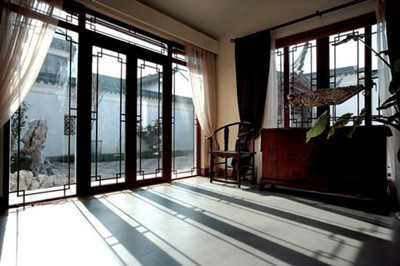中国传统室内装饰艺术的特点是总体布局对称均衡，端正稳健，而在装饰细节上崇尚自然情趣，花鸟、鱼虫等精雕细琢，富于变化，充分体现出中国传统美学精神。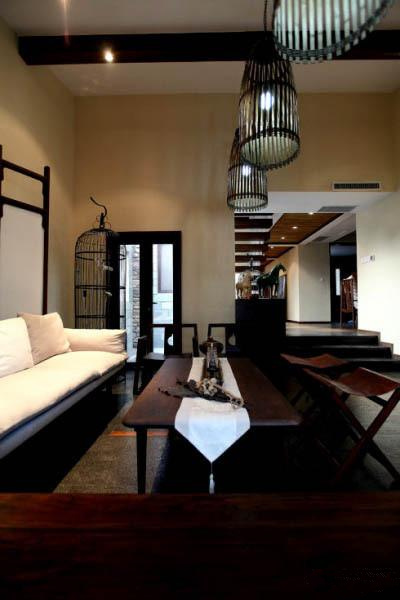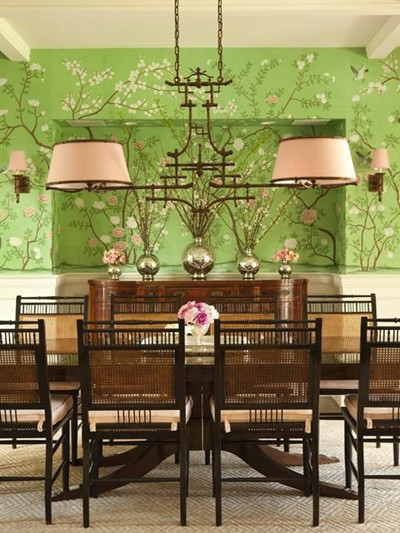新中式风格更多地使用现代技术、现代材料来表现绚丽、舒适的生活空间，同样讲究材料运用上的反差，舍弃了传统上过于复杂的机理和装饰，简化了线条，并将传统文化与现代人对生活的需求相结合，是对传统文化元素意义上的升华，也是近年来流行的家居风格。中国风并非完全意义上的复古明清，而是通过中式风格的特征，表达对清雅含蓄、端庄丰华的东方式精神境界的追求。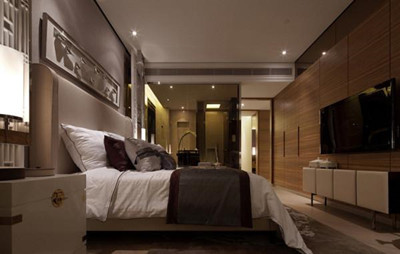`声明：本文由入驻焦点开放平台的作者撰写，除焦点官方账号外，观点仅代表作者本人，不代表焦点立场错误信息举报电话： 400-099-0099，邮箱：jubao@vip.sohu.com，或点此进行意见反馈，或点此进行举报投诉。`A B C D E F G H J K L M N P Q R S T W X Y Z
A - B - C - D - E
• A
• 鞍山
• 安庆
• 安阳
• 安顺
• 安康
• 澳门
• B
• 北京
• 保定
• 包头
• 巴彦淖尔
• 本溪
• 蚌埠
• 亳州
• 滨州
• 北海
• 百色
• 巴中
• 毕节
• 保山
• 宝鸡
• 白银
• 巴州
• C
• 承德
• 沧州
• 长治
• 赤峰
• 朝阳
• 长春
• 常州
• 滁州
• 池州
• 长沙
• 常德
• 郴州
• 潮州
• 崇左
• 重庆
• 成都
• 楚雄
• 昌都
• 慈溪
• 常熟
• D
• 大同
• 大连
• 丹东
• 大庆
• 东营
• 德州
• 东莞
• 德阳
• 达州
• 大理
• 德宏
• 定西
• 儋州
• 东平
• E
• 鄂尔多斯
• 鄂州
• 恩施
F - G - H - I - J
• F
• 抚顺
• 阜新
• 阜阳
• 福州
• 抚州
• 佛山
• 防城港
• G
• 赣州
• 广州
• 桂林
• 贵港
• 广元
• 广安
• 贵阳
• 固原
• H
• 邯郸
• 衡水
• 呼和浩特
• 呼伦贝尔
• 葫芦岛
• 哈尔滨
• 黑河
• 淮安
• 杭州
• 湖州
• 合肥
• 淮南
• 淮北
• 黄山
• 菏泽
• 鹤壁
• 黄石
• 黄冈
• 衡阳
• 怀化
• 惠州
• 河源
• 贺州
• 河池
• 海口
• 红河
• 汉中
• 海东
• 怀来
• I
• J
• 晋中
• 锦州
• 吉林
• 鸡西
• 佳木斯
• 嘉兴
• 金华
• 景德镇
• 九江
• 吉安
• 济南
• 济宁
• 焦作
• 荆门
• 荆州
• 江门
• 揭阳
• 金昌
• 酒泉
• 嘉峪关
K - L - M - N - P
• K
• 开封
• 昆明
• 昆山
• L
• 廊坊
• 临汾
• 辽阳
• 连云港
• 丽水
• 六安
• 龙岩
• 莱芜
• 临沂
• 聊城
• 洛阳
• 漯河
• 娄底
• 柳州
• 来宾
• 泸州
• 乐山
• 六盘水
• 丽江
• 临沧
• 拉萨
• 林芝
• 兰州
• 陇南
• M
• 牡丹江
• 马鞍山
• 茂名
• 梅州
• 绵阳
• 眉山
• N
• 南京
• 南通
• 宁波
• 南平
• 宁德
• 南昌
• 南阳
• 南宁
• 内江
• 南充
• P
• 盘锦
• 莆田
• 平顶山
• 濮阳
• 攀枝花
• 普洱
• 平凉
Q - R - S - T - W
• Q
• 秦皇岛
• 齐齐哈尔
• 衢州
• 泉州
• 青岛
• 清远
• 钦州
• 黔南
• 曲靖
• 庆阳
• R
• 日照
• 日喀则
• S
• 石家庄
• 沈阳
• 双鸭山
• 绥化
• 上海
• 苏州
• 宿迁
• 绍兴
• 宿州
• 三明
• 上饶
• 三门峡
• 商丘
• 十堰
• 随州
• 邵阳
• 韶关
• 深圳
• 汕头
• 汕尾
• 三亚
• 三沙
• 遂宁
• 山南
• 商洛
• 石嘴山
• T
• 天津
• 唐山
• 太原
• 通辽
• 铁岭
• 泰州
• 台州
• 铜陵
• 泰安
• 铜仁
• 铜川
• 天水
• 天门
• W
• 乌海
• 乌兰察布
• 无锡
• 温州
• 芜湖
• 潍坊
• 威海
• 武汉
• 梧州
• 渭南
• 武威
• 吴忠
• 乌鲁木齐
X - Y - Z
• X
• 邢台
• 徐州
• 宣城
• 厦门
• 新乡
• 许昌
• 信阳
• 襄阳
• 孝感
• 咸宁
• 湘潭
• 湘西
• 西双版纳
• 西安
• 咸阳
• 西宁
• 仙桃
• 西昌
• Y
• 运城
• 营口
• 盐城
• 扬州
• 鹰潭
• 宜春
• 烟台
• 宜昌
• 岳阳
• 益阳
• 永州
• 阳江
• 云浮
• 玉林
• 宜宾
• 雅安
• 玉溪
• 延安
• 榆林
• 银川
• Z
• 张家口
• 镇江
• 舟山
• 漳州
• 淄博
• 枣庄
• 郑州
• 周口
• 驻马店
• 株洲
• 张家界
• 珠海
• 湛江
• 肇庆
• 中山
• 自贡
• 资阳
• 遵义
• 昭通
• 张掖
• 中卫

1室1厅1厨1卫1阳台

1
2
3
4
5

0
1
2

1

1

0
1
2
3报名成功，资料已提交审核A B C D E F G H J K L M N P Q R S T W X Y Z
A - B - C - D - E
• A
• 鞍山
• 安庆
• 安阳
• 安顺
• 安康
• 澳门
• B
• 北京
• 保定
• 包头
• 巴彦淖尔
• 本溪
• 蚌埠
• 亳州
• 滨州
• 北海
• 百色
• 巴中
• 毕节
• 保山
• 宝鸡
• 白银
• 巴州
• C
• 承德
• 沧州
• 长治
• 赤峰
• 朝阳
• 长春
• 常州
• 滁州
• 池州
• 长沙
• 常德
• 郴州
• 潮州
• 崇左
• 重庆
• 成都
• 楚雄
• 昌都
• 慈溪
• 常熟
• D
• 大同
• 大连
• 丹东
• 大庆
• 东营
• 德州
• 东莞
• 德阳
• 达州
• 大理
• 德宏
• 定西
• 儋州
• 东平
• E
• 鄂尔多斯
• 鄂州
• 恩施
F - G - H - I - J
• F
• 抚顺
• 阜新
• 阜阳
• 福州
• 抚州
• 佛山
• 防城港
• G
• 赣州
• 广州
• 桂林
• 贵港
• 广元
• 广安
• 贵阳
• 固原
• H
• 邯郸
• 衡水
• 呼和浩特
• 呼伦贝尔
• 葫芦岛
• 哈尔滨
• 黑河
• 淮安
• 杭州
• 湖州
• 合肥
• 淮南
• 淮北
• 黄山
• 菏泽
• 鹤壁
• 黄石
• 黄冈
• 衡阳
• 怀化
• 惠州
• 河源
• 贺州
• 河池
• 海口
• 红河
• 汉中
• 海东
• 怀来
• I
• J
• 晋中
• 锦州
• 吉林
• 鸡西
• 佳木斯
• 嘉兴
• 金华
• 景德镇
• 九江
• 吉安
• 济南
• 济宁
• 焦作
• 荆门
• 荆州
• 江门
• 揭阳
• 金昌
• 酒泉
• 嘉峪关
K - L - M - N - P
• K
• 开封
• 昆明
• 昆山
• L
• 廊坊
• 临汾
• 辽阳
• 连云港
• 丽水
• 六安
• 龙岩
• 莱芜
• 临沂
• 聊城
• 洛阳
• 漯河
• 娄底
• 柳州
• 来宾
• 泸州
• 乐山
• 六盘水
• 丽江
• 临沧
• 拉萨
• 林芝
• 兰州
• 陇南
• M
• 牡丹江
• 马鞍山
• 茂名
• 梅州
• 绵阳
• 眉山
• N
• 南京
• 南通
• 宁波
• 南平
• 宁德
• 南昌
• 南阳
• 南宁
• 内江
• 南充
• P
• 盘锦
• 莆田
• 平顶山
• 濮阳
• 攀枝花
• 普洱
• 平凉
Q - R - S - T - W
• Q
• 秦皇岛
• 齐齐哈尔
• 衢州
• 泉州
• 青岛
• 清远
• 钦州
• 黔南
• 曲靖
• 庆阳
• R
• 日照
• 日喀则
• S
• 石家庄
• 沈阳
• 双鸭山
• 绥化
• 上海
• 苏州
• 宿迁
• 绍兴
• 宿州
• 三明
• 上饶
• 三门峡
• 商丘
• 十堰
• 随州
• 邵阳
• 韶关
• 深圳
• 汕头
• 汕尾
• 三亚
• 三沙
• 遂宁
• 山南
• 商洛
• 石嘴山
• T
• 天津
• 唐山
• 太原
• 通辽
• 铁岭
• 泰州
• 台州
• 铜陵
• 泰安
• 铜仁
• 铜川
• 天水
• 天门
• W
• 乌海
• 乌兰察布
• 无锡
• 温州
• 芜湖
• 潍坊
• 威海
• 武汉
• 梧州
• 渭南
• 武威
• 吴忠
• 乌鲁木齐
X - Y - Z
• X
• 邢台
• 徐州
• 宣城
• 厦门
• 新乡
• 许昌
• 信阳
• 襄阳
• 孝感
• 咸宁
• 湘潭
• 湘西
• 西双版纳
• 西安
• 咸阳
• 西宁
• 仙桃
• 西昌
• Y
• 运城
• 营口
• 盐城
• 扬州
• 鹰潭
• 宜春
• 烟台
• 宜昌
• 岳阳
• 益阳
• 永州
• 阳江
• 云浮
• 玉林
• 宜宾
• 雅安
• 玉溪
• 延安
• 榆林
• 银川
• Z
• 张家口
• 镇江
• 舟山
• 漳州
• 淄博
• 枣庄
• 郑州
• 周口
• 驻马店
• 株洲
• 张家界
• 珠海
• 湛江
• 肇庆
• 中山
• 自贡
• 资阳
• 遵义
• 昭通
• 张掖
• 中卫• 手机• 分享
• 设计
免费设计
• 计算器
装修计算器
• 入驻
合作入驻
• 联系
联系我们
• 置顶
返回顶部